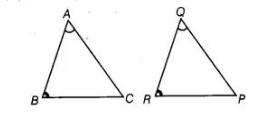# In ΔABC and ΔPQR,`
Question:

In ΔABC and ΔPQR, ∠A = ∠Q and ∠B =∠R. Which side of ΔPQR should be equal to side AB of ΔABC, so that the two triangles are congruent? Give

We have given, in $\triangle A B C$ and $\triangle P Q R, \angle A=\angle Q$ and $\angle B=\angle R$